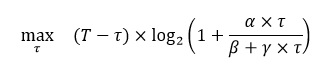# Can CVX solve this kind of function {x-log(1+0.01*x/(x+1))}

My code is as follow：

``````cvx_begin
variable x;
minimize(x-1000*log(1+0.01*x/(x+1));
subject to
x>0;
cvx_end
``````

Then it reported:

Disciplined convex programming error:
Cannot perform the operation: {real affine} ./ {real affine}

log(1+x/y) is a kind of formation of Information entropy，and my obj function is concave. Is there any ways to rewrite this object function ? Thank you.

What if you write it as `x-1000*log(1.01-0.01*inv_pos((x+1)))`. I’m not sure this is correct cvx syntax, I just mean transform the expression like this.

1 Like

Well,This is a problem. I just don’t know what kind of expression it should be converted into.And `x-1000*log(1+0.01*x*inv_pos(x+1))` reported another error：

Cannot perform the operation: {positive constant} ./ {real affine}

Or I don’t know if it can solve this problem just like this expression

All I know is that my expression is equivalent to yours and conic representable. I’m not sure it implies it also works in cvx but if not it should be only a matter of me making a syntax error.

@Michal_Adamaszek 's solution is correct, and is accepted and solved by CVX.

``````cvx_begin
variable x
minimize(x-1000*log(1.01-0.01*inv_pos((x+1))))
subject to
x>0
cvx_end
``````

@04014540 Can you show us the complete program you ran which triggered the error message `Cannot perform the operation: {positive constant} ./ {real affine}` ?

Sorry,I just found my mistake.@Michal_Adamaszek 's solution is right.Thank you so much and I can use a model that similar to this to solve the problem about Information entropy.Best wish to you.

Good afternoon. I have met a relevant about Information entropy question like log(1+1/x)<a. Would you mind send your problem and the corresponding solution to me? It might be a great honor for me to receive your reply. Many thanks!

``````maximize(Mn-mu_n*(1+exp(-an*(gnx*(P(1)+P(2))-bn))))
subject to
R1 = log2(1+(P(1)*hix)*inv_pos(P(2)*hix)+Ni);
R2 = log2(1+(P(2)*hjx)*inv_pos(Nj));
R3 = log2(1+(P(1)*hjx)*inv_pos((P(2)*hjx)+Nj));
R1 >= R_min;
R2 >= R_min;
R3 >= R1+R2;
``````

Getting Error
Cannot perform the operation: {real affine} .* {convex}

Error in * (line 36)
z = feval( oper, x, y );

Error in nov22 (line 41)
R1 = log2(1+(P(1)*hix)*inv_pos(P(2)*hix)+Ni);

I provided an answer to your question at Disciplined convex programming error: Cannot perform the operation: {real affine} .* {convex} .which I think addresses your question here.

hi
my problem as follows:where t is optimization variable and t <=T and alpha, beta and gamma >0 and real number.
how can rewrite T * log (1+a *x / (b + g *x)) in cvx?
for b and g in the de-numerator, I can’t use the same method

Your remaining piece looks something like `log(1+0.01*x/(x+1))` . Make the necessary adaptation. It’s your problem, so you should put in at least some of the effort.

I can’t use this method because I can’t reform the de-numerator to (1+x)
i know a *x / (b + g *x) is can be concave and convex in the domain and DCP can’t perform what is… and I must use inv_pos(.) to define for DCP.
i used a x * inv_pos(b + g * x) but give :Cannot perform the operation: {real affine} . {convex}
i think a *x term must import to inv_pos() function or rewrite a *x / (b + g *x) as affine function for t and use inv_pos() but I can’t…

Let `aa = a/g; bb=b/g;`

`log(1+aa*t/(bb+t)) = log(1+aa-aa*bb*inv_pos(bb+t))`, the latter of which complies with CVX’s rules.

So this generalizes (adapts) the reformulation provided above by @Michal_Adamaszek to `bb` not necessarily equal to 1.

2 Likes

NICE NICE NICE mark…